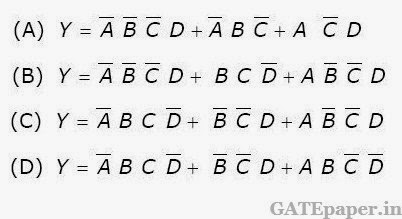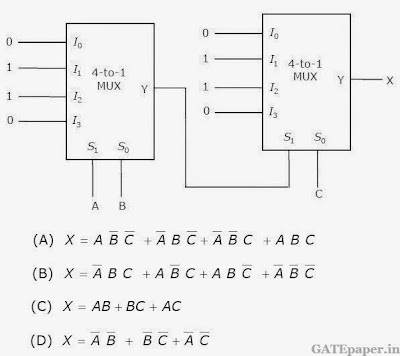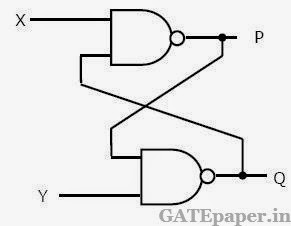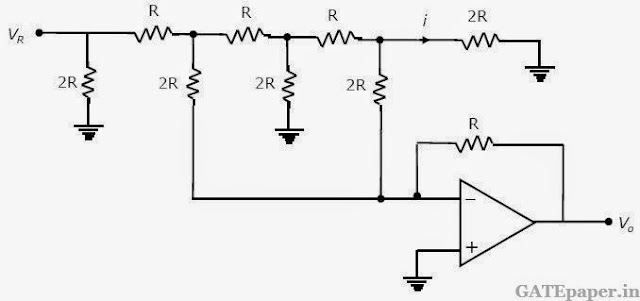### GATE 2007 ECE Video Solution on Digital Circuits (Digital Electronics)

1. X = 01110 and Y = 11001 are two 5 bit binary numbers represented in 2’s compliement format. The sum of X and Y represented in 2’s compliment format using 6 bits is
a. 100111
b. 001000
c. 000111
d. 101001

2. The Boolean function Y = AB + CD is to be realized using only 2 input NAND gates. The minimum number of gates required is
a. 2
b. 3
c. 4
d. 5

3. The following Boolean expression can be minimized to4. The circuit diagram of a standard TTL NOT gate is shown in the figure. When Vi = 2.5 volts, the modes of operation of the transistor will bea. Q1 reverse active, Q2 normal active, Q3 saturation and Q4 cut-off
b. Q1 reverse active, Q2 saturation, Q3 saturation and Q4 cut-off
c. Q1 normal active, Q2 cut-off, Q3 cut-off and Q4 saturation
d. Q1 saturation, Q2 saturation, Q3 saturation and Q4 normal active

5. In the following circuit X is given by6. In the following circuit, binary values were applied to the inputs X and Y inputs of the NAND latch shown in the figure in the sequence indicated below:
X = 0, Y = 1; X = 0, Y = 0; X = 1, Y = 1;The corresponding stable P, Q outputs will be7. For the circuit shown, the counter state (Q1Q0) follows the sequencea. 00, 01, 10, 11, 00…..
b. 00, 01, 10, 00, 01…..
c. 00, 01, 11, 00, 01…..
d. 00, 10, 11, 00, 10…..

8. An 8255 chip is interfaced to an 8085 microprocessor system as an I/O mapped I/O as shown in the figure. The address lines A0 and A1 of the 8085 are used by the 8255 chip to decode internally its three ports and the control register. The address lines A0 to A7 as well as the IO/M’ signal are used for address decoding. The range of addressees for which the 8255 chip would get selected isa. F8H – FBH
b. F8H – FCH
c. F8H – FFH
d. F0H – F7H

An 8085 assembly language program is given below.
Line 1 MVI A, B5H
2 MVI B, 0EH
3 XRI 69H
5 ANI 9BH
6 CPI 9FH
7 STA 3010H
8 HLT

9. The contents of the accumulator just after execution of the ADD instruction in line 4 will
be
a. C3H
b. EAH
c. DCH
d. 69H

10. After execution of line 7 of the program, the status of the CY and Z flags will be ….. respectively.
a. 0, 0
b. 0, 1
c. 1, 0
d. 1, 1

In the Digital to analog converter circuit shown in the figure below, VR = 10 volts and R = 10 kΩ.11. The current i is
a. 31.25 µA
b. 62.5 µA
c. 125 µA
d. 250 µA

12. The voltage Vo is
a. – 0.781 volts
b. – 1.562 volts
c. – 3.125 volts
d. – 6.250 volts
Solution (11 & 12) : https://www.youtube.com/watch?v=ByhKwkrj-qs